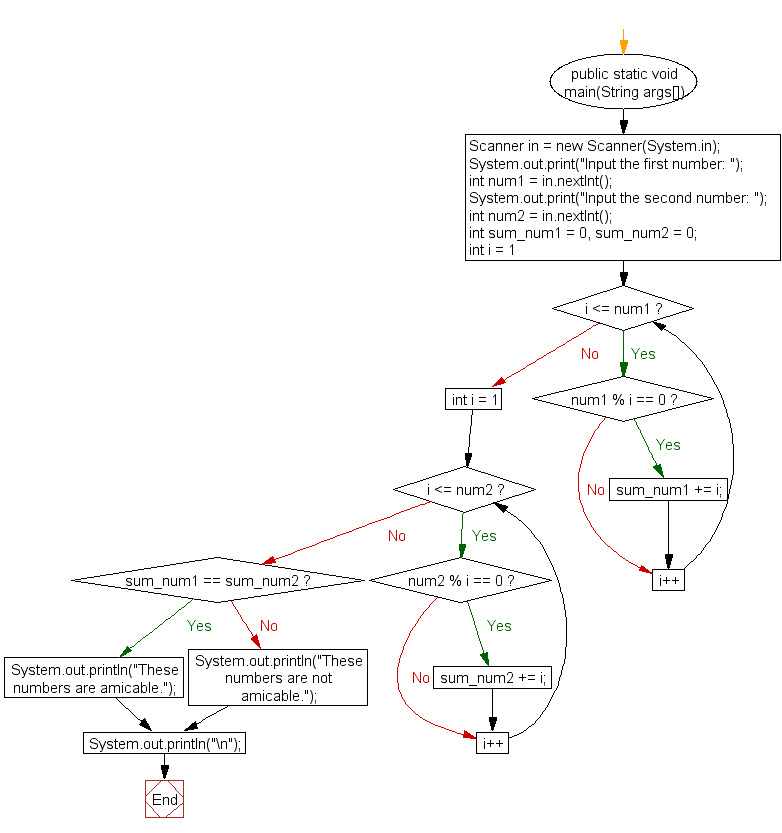﻿ Java exercises: Check two numbers are Amicable numbers or not - w3resource# Java Exercises: Check two numbers are Amicable numbers or not

## Java Numbers: Exercise-16 with Solution

Write a Java program to check two numbers are Amicable numbers or not.

Amicable numbers are two different numbers so related that the sum of the proper divisors of each is equal to the other number.
The first ten amicable pairs are: (220, 284), (1184, 1210), (2620, 2924), (5020, 5564), (6232, 6368), (10744, 10856), (12285, 14595), (17296, 18416), (63020, 76084), and (66928, 66992).

Test Data
Input the first number: 220
Input the second number: 284

Pictorial Presentation:Sample Solution:

Java Code:

``````import java.util.Scanner;

public class Example16
{
public static void main(String args[]) {
Scanner in = new Scanner(System.in);
System.out.print("Input the first number: ");
int num1 = in.nextInt();
System.out.print("Input the second number: ");
int num2 = in.nextInt();
int sum_num1 = 0, sum_num2 = 0;
for (int i = 1; i <= num1; i++) {
if (num1 % i == 0)
sum_num1 += i;
}
for (int i = 1; i <= num2; i++) {
if (num2 % i == 0)
sum_num2 += i;
}
if (sum_num1 == sum_num2)
System.out.println("These numbers are amicable.");
else
System.out.println("These numbers are not amicable.");
System.out.println("\n");
}
}
```
```

Sample Output:

```Input the first number: 220
Input the second number: 284
These numbers are amicable.
```

Flowchart:Java Code Editor:

What is the difficulty level of this exercise?

﻿

## Java: Tips of the Day

Array vs ArrayLists:

The main difference between these two is that an Array is of fixed size so once you have created an Array you cannot change it but the ArrayList is not of fixed size. You can create instances of ArrayLists without specifying its size. So if you create such instances of an ArrayList without specifying its size Java will create an instance of an ArrayList of default size.

Once an ArrayList is full it re-sizes itself. In fact, an ArrayList is internally supported by an array. So when an ArrayList is resized it will slow down its performance a bit as the contents of the old Array must be copied to a new Array.

At the same time, it's compulsory to specify the size of an Array directly or indirectly while creating it. And also Arrays can store both primitives and objects while ArrayLists only can store objects.

Ref: https://bit.ly/3o8L2KH